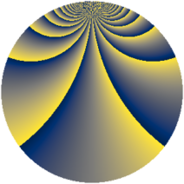# Properties

 Label 2736.2.frLevel $2736$ Weight $2$ Character orbit 2736.fr Rep. character $\chi_{2736}(895,\cdot)$ Character field $\Q(\zeta_{18})$ Dimension $720$ Sturm bound $960$

# Related objects

## Defining parameters

 Level: $$N$$ $$=$$ $$2736 = 2^{4} \cdot 3^{2} \cdot 19$$ Weight: $$k$$ $$=$$ $$2$$ Character orbit: $$[\chi]$$ $$=$$ 2736.fr (of order $$18$$ and degree $$6$$) Character conductor: $$\operatorname{cond}(\chi)$$ $$=$$ $$684$$ Character field: $$\Q(\zeta_{18})$$ Sturm bound: $$960$$

## Dimensions

The following table gives the dimensions of various subspaces of $$M_{2}(2736, [\chi])$$.

Total New Old
Modular forms 2952 720 2232
Cusp forms 2808 720 2088
Eisenstein series 144 0 144

## Trace form

 $$720q - 36q^{9} + O(q^{10})$$ $$720q - 36q^{9} - 36q^{33} - 36q^{41} + 360q^{49} + 108q^{73} + 36q^{81} - 108q^{97} + O(q^{100})$$

## Decomposition of $$S_{2}^{\mathrm{new}}(2736, [\chi])$$ into newform subspaces

The newforms in this space have not yet been added to the LMFDB.

## Decomposition of $$S_{2}^{\mathrm{old}}(2736, [\chi])$$ into lower level spaces

$$S_{2}^{\mathrm{old}}(2736, [\chi]) \cong$$ $$S_{2}^{\mathrm{new}}(684, [\chi])$$$$^{\oplus 3}$$